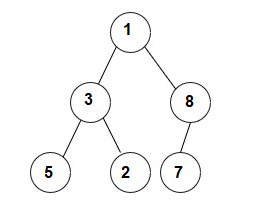New update is available. Click here to update.

# Node Level

Last Updated: 2 Dec, 2020
Difficulty: Easy

## PROBLEM STATEMENT

#### Note:

``````Consider root to be at level 1. It is guaranteed that all the nodes in the binary tree have distinct values.
``````
##### Input Format:
``````The first line contains an integer 'T' which denotes the number of test cases or queries to be run.

The first line of each test case contains elements of the tree in the level order form. The line consists of values of nodes separated by a single space. In case a node is null, we take -1 in its place.

The second line of each test case contains one integer ‘M’ denoting the node whose level has to be calculated.

For example, the input for the tree depicted in the below image.
````````````1
3 8
5 2 7 -1
-1 -1 -1 -1 -1 -1
``````
##### Explanation :
``````Level 1 :
The root node of the tree is 1

Level 2 :
Left child of 1 = 3
Right child of 1 = 8

Level 3 :
Left child of 3 = 5
Right child of 3 = 2
Left child of 8 =7
Right child of 8 =  null (-1)

Level 4 :
Left child of 5 = null (-1)
Right child of 5 = null (-1)
Left child of 2 = null (-1)
Right child of 2 = null (-1)
Left child of 7 = null (-1)
Right child of 7 = null (-1)

The first not-null node(of the previous level) is treated as the parent of the first two nodes of the current level. The second not-null node (of the previous level) is treated as the parent node for the next two nodes of the current level and so on.
The input ends when all nodes at the last level are null(-1).
``````
##### Note :
``````The above format was just to provide clarity on how the input is formed for a given tree.

The sequence will be put together in a single line separated by a single space. Hence, for the above-depicted tree, the input will be given as:
1 3 8 5 2 7 -1 -1 -1 -1 -1 -1 -1
``````
##### Output Format:
``````For each test case, return a single line containing the level specified by the node. If the node is not present, return 0.

The output of each test case will be printed in a separate line.
``````
##### Note :
``````You do not need to print anything, it has already been taken care of. Just implement the given function.
``````
##### Constraints:
``````1 <= T <= 5
0 <= N <= 3000
0 <= node data <= 10^9

Time Limit: 1 sec
``````